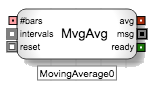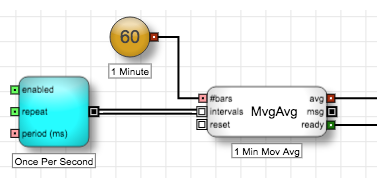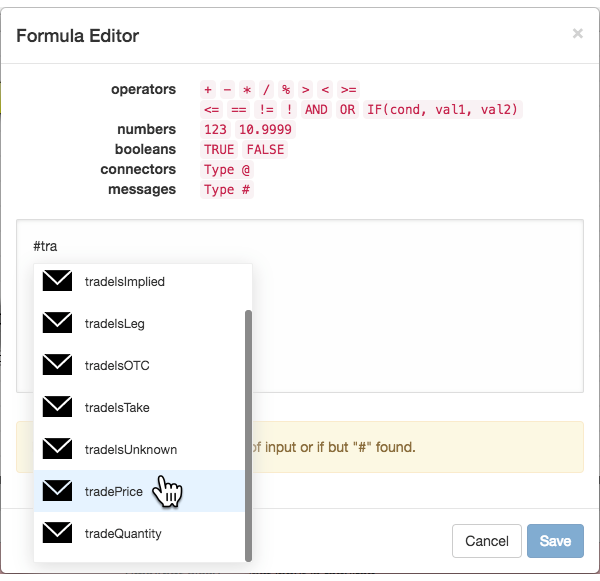Discrete blocks

# Moving Average blockThe Moving Average block maintains an internal collection of numeric values (such as LTP), as defined by its internal formula, and outputs the average of the most recent user-specified number of values (#bars). Every time the block receives a message on its Intervals port, it performs the following actions:

1. Updates its internal collection of the user-specified value.
2. Calculates the average of the #bars most-recent number of values and sends the average through its avg output port.
3. Passes the original intervals message unchanged through its msg output port.
4. Sends TRUE through its ready port after the initial #bars number of messages to indicate that the avg output includes the desired number of data points.

Example The Moving Average block receives an empty discrete message from the Generator block every second. The number passed into the #bars input port specifies that the moving average calculation should be based on the 60 values that are collected over one minute. Each time it receives an input message through its intervals ports, the block adds a new value to its collection and recalculates its moving average based on the most recent 60 values and outputs that average through its avg port to be used by downstream logic. Once the Moving Average block receives 60 messages, it changes its ready port to TRUE, which downstream logic can use to determine whether the avg output contains enough data points.Formula to calculate the moving average of the last-traded price (LTP)You can use the following ports to control whether your algo uses the moving average value:

• ready: This port can be used to determine whether the block has collected sufficient number of bars to satisfy the #bars input. If the block does not have sufficient number of bars, the ready port will output False. When the block accumulates sufficient number of bars, the ready port will output TRUE.

• reset: This port can be used to force the Moving Average block to delete its internal data collection and reset the avg to a NaN (Not A Number) when a discrete event message triggers the reset port.

## Block properties

To customize the block, update the Block Properties.

Property Description
Name Name to display beneath the block on the ADL canvas
Formula

Equation used to calculate the value of the block

The edit link opens the Formula Editor.